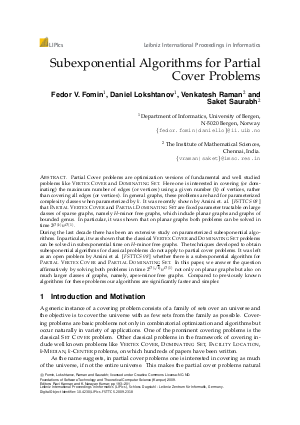Document# Subexponential Algorithms for Partial Cover Problems

### Authors Fedor V. Fomin, Daniel Lokshtanov, Venkatesh Raman, Saket Saurabh## File

LIPIcs.FSTTCS.2009.2318.pdf
• Filesize: 196 kB
• 9 pages

## Cite As

Fedor V. Fomin, Daniel Lokshtanov, Venkatesh Raman, and Saket Saurabh. Subexponential Algorithms for Partial Cover Problems. In IARCS Annual Conference on Foundations of Software Technology and Theoretical Computer Science. Leibniz International Proceedings in Informatics (LIPIcs), Volume 4, pp. 193-201, Schloss Dagstuhl - Leibniz-Zentrum für Informatik (2009)
https://doi.org/10.4230/LIPIcs.FSTTCS.2009.2318

## Abstract

Partial Cover problems are optimization versions of fundamental and well studied problems like {\sc Vertex Cover} and {\sc Dominating Set}. Here one is interested in covering (or dominating) the maximum number of edges (or vertices) using a given number ($k$) of vertices, rather than covering all edges (or vertices). In general graphs, these problems are hard for parameterized complexity classes when parameterized by $k$. It was recently shown by Amini et. al. [{\em FSTTCS 08}\,] that {\sc Partial Vertex Cover} and {\sc Partial Dominating Set} are fixed parameter tractable on large classes of sparse graphs, namely $H$-minor free graphs, which include planar graphs and graphs of bounded genus. In particular, it was shown that on planar graphs both problems can be solved in time $2^{\cO(k)}n^{\cO(1)}$.
##### Keywords
• Partial cover problems
• parameterized complexity
• subexponential time algorithms
• irrelevant vertex technique

## Metrics

• Access Statistics
• Total Accesses (updated on a weekly basis)
0